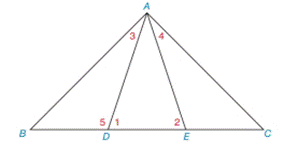Chapter 2.4, Problem 20E### Elementary Geometry for College St...

6th Edition
Daniel C. Alexander + 1 other
ISBN: 9781285195698

#### Solutions

Chapter
Section### Elementary Geometry for College St...

6th Edition
Daniel C. Alexander + 1 other
ISBN: 9781285195698
Textbook Problem
1 views

# Given: Δ A B C with B − D − C − E m ∠ 1 = 2 x m ∠ 3 = x Find: m ∠ B in terms of xTo determine

To find:

The value of mB.

Explanation

Given:

The given statement is,

m1=2xm3=x

Figure (1)

Properties:

(1) In a triangle, the sum of the measures of the interior angles is 180°.

(2) Supplementary angles add up to 180°.

Calculation:

The given statement is,

m1=2xm3=x

1 and 5 are supplementary angles.

m1+m5=180°(1)

Substitute 2x for m1 in equation (1)

### Still sussing out bartleby?

Check out a sample textbook solution.

See a sample solution

#### The Solution to Your Study Problems

Bartleby provides explanations to thousands of textbook problems written by our experts, many with advanced degrees!

Get Started

#### 103/2

Applied Calculus for the Managerial, Life, and Social Sciences: A Brief Approach

#### Subtract: 2301506

Elementary Technical Mathematics

#### JacobianState the definition of the Jacobian.

Calculus: Early Transcendental Functions (MindTap Course List)

#### Using for |x| < 1,

Study Guide for Stewart's Multivariable Calculus, 8th